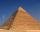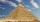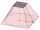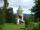# Unit conversion + pyramid - math problems

#### Number of problems found: 11

• Pyramid of GizaThe Great Pyramid of Giza has the shape of a regular quadrangular pyramid. The base edge is 227 m long and 140 m high. What is the weight of the stone needed to build this pyramid, if the weight of 1 m3 of stone is 2.5 t?
• Pyramid a+hCalculate the volume and surface area of the pyramid on the edge and height a = 26 cm. h = 3 dm.
• Cheops pyramidThe Pyramid of Cheops is a pyramid with a square base with a side of 233 m and a height of 146.6 m. It made from limestone with a density of 2.7 g/cm3. Calculate the amount of stone in tons. How many trains with 30 twenty tons wagons carry the stone?
• Truncated pyramidHow many cubic meters is the volume of a regular four-sided truncated pyramid with edges one meter and 60 cm and high 250 mm?
• Tetrahedral pyramidCalculate the volume and surface area of a regular tetrahedral pyramid, its height is \$b cm and the length of the edges of the base is 6 cm.
• Truncated pyramidThe concrete pedestal in the shape of a regular quadrilateral truncated pyramid has a height of 12 cm, the pedestal edges have lengths of 2.4 and 1.6 dm. Calculate the surface of the base.The quadrilateral pyramid has a rectangular base of 24 cm x 3.2dm and a body height of 0.4m. Calculate its volume and surface area.
• Runcated pyramid teapotThe 35 cm high teapot has the shape of a truncated pyramid with the length of the edge of the lower square base a=50 cm and with the edges of the rectangular base b: 20 cm and c: 30 cm. How many liters of water will fit in the teapot?
• Heptagonal pyramidA hardwood for a column is in the form of a frustum of a regular heptagonal pyramid. The lower base edge is 18 cm and the upper base of 14 cm. The altitude is 30 cm. Determine the weight in kg if the density of the wood is 10 grams/cm3.
• Roof 8How many liters of air are under the roof of tower which has the shape of a regular six-sided pyramid with a 3,6-meter-long bottom edge and a 2,5-meter height? Calculate the supporting columns occupy about 7% of the volume under the roof.
• The Scout TentThe Scout Tent has a rectangular wooden underlay with dimensions of 220 cm and 150 cm. How much canvas is needed for a 170 cm high of pyramid roof?

We apologize, but in this category are not a lot of examples.
Do you have an interesting mathematical word problem that you can't solve it? Submit a math problem, and we can try to solve it.

We will send a solution to your e-mail address. Solved examples are also published here. Please enter the e-mail correctly and check whether you don't have a full mailbox.

Please do not submit problems from current active competitions such as Mathematical Olympiad, correspondence seminars etc...

Unit conversion - math word problems. Pyramid Problems.